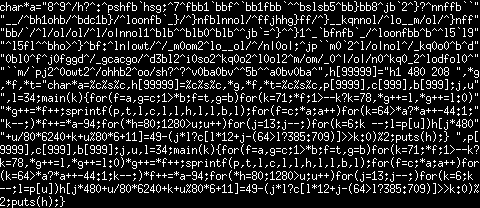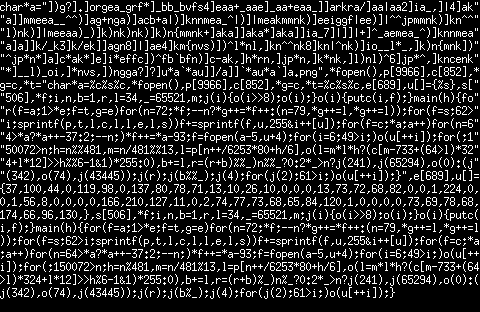back

# Image quines

These are 2 code-golfed C programs which print their own source code (the definition of a quine, really). However, these are no regular quines: instead of simply printing their source code as text, they instead print a screenshot of themselves, as would be seen by viewing their source code in `xterm`.

There's 2 of them because I had 2 different targets: the shortest file whose output format could reasonably be called an image, and the shortest file whose output was a PNG. The output format for the first one was PBM, simply because it's the simplest format I knew of that was actually recognized by most image viewers.

The PBM quine's code is:

```char*a="8^9^/h?^:^pshfb`hsg;^7^fbb1`bbf^`bb1fbb`^^bslsb5^bb}bb8^jb`2^}?^nnffb``"
"__/^bh1ohb/^bdc1b}/^loonfb`_}/^}nfblnnol/^ffjhhg}ff/^}__kqnnol/^lo__m/ol/^}nff"
"bb/`/^l/ol/ol/^l/o|nnol1^blb^^blb0^blb^^jb`=^}^^}1^_`bfnfb`_/^loonfbb^b^^l5`l9"
"^l5fl^^bho>^}^bf:^ln|owt/^/_m0om2^lo__ol/^/n|0o|;^jp``m0`2^l/o|nol^/_kq0o0^b^d"
"0bl0^f^j0fggd^/_gcacgo/^d3bl2^i0so2^kq0o2^l0ol2^m/om/_0^|/o|/n0^kq0_2^lodfol0^"
"``m/`pj2^0owt2^/ohhb2^oo/sh?^?^v0ba0bv^^5b^^a0bv0ba^",h="h1 480 208 ",*g
,*f,*t="char*a=%c%s%c,h=%c%s%c,*g,*f,*t=%c%s%c,p,c,b;j,u"
",l=34;main(k){for(f=a,g=c;1>*b;f=t,g=b)for(k=71;*f;1>--k?k=78,*g++=l,*g++=l:0)"
"*g++=*f++;sprintf(p,t,l,c,l,l,h,l,l,b,l);for(f=c;*a;a++)for(k=64>*a?*a++-44:1;"
"k--;)*f++=*a-94;for(*h=80;1280>u;u++)for(j=13;j--;)for(k=6;k --;l=p[u])h[j*480"
"+u/80*6240+k+u%80*6+11]=49-(j*l?c[l*12+j-(64>l?385:709)]>>k:0)%2;puts(h);} ",p[
9999],c,b;j,u,l=34;main(k){for(f=a,g=c;1>*b;f=t,g=b)for(k=71;*f;1>--k?
k=78,*g++=l,*g++=l:0)*g++=*f++;sprintf(p,t,l,c,l,l,h,l,l,b,l);for(f=c;*a;a++)for
(k=64>*a?*a++-44:1;k--;)*f++=*a-94;for(*h=80;1280>u;u++)for(j=13;j--;)for(k=6;k
--;l=p[u])h[j*480+u/80*6240+k+u%80*6+11]=49-(j*l?c[l*12+j-(64>l?385:709)]>>k:0)%
2;puts(h);}
```
imgquine_pbm.c (1225 bytes)

And it produces the following image:The PNG version's source code is:

```char*a="])g?].]orgea_grf*]_bb_bvfs4]eaa+_aae]_aa+eaa_]]arkra/]aa|aa2]ia_,]|4]ak"
"a]]mmeea__^^)]ag+nga)]acb+a|)]knnmea_^|)]|meakmmnk)]eeiggf|ee)]|^^jpmmnk)]kn^^"
"l)nk)]|meeaa)_)]k)nk)nk)]k)n{mmnk+]aka]]aka*]aka]]ia_7]|]]|+]^_aemea_^)]knnmea"
"a]a]]k/_k3]k/ek]]agn8]|]ae4]km{nvs)])^l*nl,]kn^^nk8]kn|^nk)]io__l*_,]k)n{mnk])"
"^jp*n*]a]c*ak*]e]i*effc])^fb`bfn)]c-ak,]h*rn,]jp*n,]k*nk,]l)nl)^6]jp*^,]kncenk"
"*]__l)_oi,]*nvs,])ngga?]?]u*a`*au]]/a]]`*au*a`]a.png",*fopen(),p,c,*
g=c,*t="char*a=%c%s%c,*fopen(),p,c,*g=c,*t=%c%s%c,e,u[]={%s},s["
"506],*f;i,n,b=1,r,l=34,_=65521,m;j(i){o(i>>8);o(i);}o(i){putc(i,f);}main(h){fo"
"r(f=a;1>*e;f=t,g=e)for(n=72;*f;--n?*g++=*f++:(n=79,*g++=l,*g++=l));for(f=s;62>"
"i;sprintf(p,t,l,c,l,l,e,l,s))f+=sprintf(f,u,255&i++[u]);for(f=c;*a;a++)for(n=6"
"4>*a?*a++-37:2;--n;)*f++=*a-93;f=fopen(a-5,u+4);for(i=6;49>i;)o(u[++i]);for(;1"
"50072>n;h=n%%481,m=n/481%%13,l=p[n++/6253*80+h/6],o(l=m*l*h?(c[m-733+(64>l)*32"
"4+l*12]>>h%%6-1&1)*255:0),b+=l,r=(r+b)%%_)n%%_?0:2*_>n?j(241),j(65294),o(0):(j"
"(342),o(74),j(43445));j(r);j(b%%_);j(4);for(j(2);61>i;)o(u[++i]);}",e,u[]=
{37,100,44,0,119,98,0,137,80,78,71,13,10,26,10,0,0,0,13,73,72,68,82,0,0,1,224,0,
0,1,56,8,0,0,0,0,166,210,127,11,0,2,74,77,73,68,65,84,120,1,0,0,0,0,73,69,78,68,
174,66,96,130,},s,*f;i,n,b=1,r,l=34,_=65521,m;j(i){o(i>>8);o(i);}o(i){putc(
i,f);}main(h){for(f=a;1>*e;f=t,g=e)for(n=72;*f;--n?*g++=*f++:(n=79,*g++=l,*g++=l
));for(f=s;62>i;sprintf(p,t,l,c,l,l,e,l,s))f+=sprintf(f,u,255&i++[u]);for(f=c;*a
;a++)for(n=64>*a?*a++-37:2;--n;)*f++=*a-93;f=fopen(a-5,u+4);for(i=6;49>i;)o(u[++
i]);for(;150072>n;h=n%481,m=n/481%13,l=p[n++/6253*80+h/6],o(l=m*l*h?(c[m-733+(64
>l)*324+l*12]>>h%6-1&1)*255:0),b+=l,r=(r+b)%_)n%_?0:2*_>n?j(241),j(65294),o(0):(
j(342),o(74),j(43445));j(r);j(b%_);j(4);for(j(2);61>i;)o(u[++i]);}
```
imgquine_png.c (1849 bytes)

And the output is:Note that you'll get a lot of warnings when trying to compile this. In the future, some of those warnings might even get upgraded to errors. So if you're reading this from the future, then know that the last compilers this was tested with were GCC 10.2 and Clang 10.0.

So here's how it works. We'll start with the PBM one, as the PNG version is pretty much an extension of it. `char* a` is the font, encoded as RLE with one set of characters acting as repeat counts and another set acting as actual data. The data is encoded as 5 bits per character, with each character giving one scanline of one letter of the font. The font is 6x13, but the first row and leftmost column of each character are blank, so we only need to encode a 5x12 region for each character. Also, the font doesn't cover the entire printable ASCII range, only what was needed to represent the rest of the source code. This created a bit of a dilemma, as some characters were only needed because they were used by the description of other characters in the font. I ended up running a small brute-force search for the best parameters (i.e. ones which required the fewest extra bytes to represent).

(TODO: more explanation here)

Now that we know how the PBM one works, here's what I needed to change to make it output PNGs. I got around PNG's compression by simply encoding the image data as a sequence of raw (or literal, or non-compressed) blocks. Deflate (the compression algorithm used in PNG) supports them to allow for minimal overhead when trying to compress already-compressed data: it only requires a 5-byte header to write up to 65KB of data to the output stream. However, there's nothing that checks whether the image data inside a PNG is actually well-compressed, so we can get away with encoding the entire uncompressed image data as raw blocks. This of course goes against the entire point of the PNG file format, but I'm not going to implement an actual compressor inside a quine.

There are still 2 obstacles that make the PNG variant significantly more complicated than the PBM variant: checksums. The Deflate stream includes an Adler-32 checksum, and the PNG file format mandates a CRC-32 checksum too. Adler-32 uses integer arithmetic and is much shorter to implement in plain C than CRC-32 with its polynomial rings over GF(2). So we just update the Adler-32 checksum as we compute the pixels of image data. For the CRC-32 sum, however, we can cheat: the "32" in its name signifies that the checksum itself is only 32 bits long. And as we all know, 32 bits can only encode one of around 4 billion values. So we can simply hardcode the CRC in our program, and generate random variations of the quine until one of them has the right CRC.

Lastly, I want to thank a few friends who provided invaluable help while golfing this, and without whose support I wouldn't have finished this at all: